Related Articles

# Class 10 NCERT Solutions- Chapter 13 Surface Areas And Volumes – Exercise 13.2

• Difficulty Level : Medium
• Last Updated : 04 Mar, 2021

### Question 1. A solid is in the shape of a cone standing on a hemisphere with both their radii being equal to 1 cm and the height of the cone is equal to its radius. Find the volume of the solid in terms of π.

Solution:

Given:

Attention reader! All those who say programming isn't for kids, just haven't met the right mentors yet. Join the  Demo Class for First Step to Coding Coursespecifically designed for students of class 8 to 12.

The students will get to learn more about the world of programming in these free classes which will definitely help them in making a wise career choice in the future.

Height of cone (h)= 1 cm

Radius of hemisphere (r) = 1 cm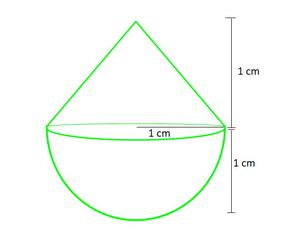Total Volume = Volume of cone + Volume of Hemisphereπr2h +πr3πr2(h+2r)× π × 1 × 1 × (1+2)

= π cm3

### Question 2. Rachel, an engineering student, was asked to make a model shaped like a cylinder with two cones attached at its two ends by using a thin aluminum sheet. The diameter of the model is 3 cm and its length is 12 cm. If each cone has a height of 2 cm, find the volume of air contained in the model that Rachel made. (Assume the outer and inner dimensions of the model to be nearly the same.)

Solution:

Given:

Radius of cone and cylinder (r) =cm

Height of cone (h) = 2 cm

Height of cylinder (H) = 12- (2+2) = 8 cm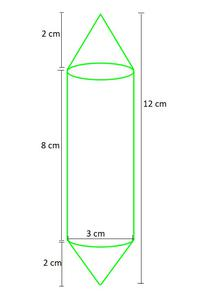Total Volume = Volume of two cones + Volume of cylinder

=πr2h +πr2h + πr2Hπr2h + πr2H

= πr2(()h+H)(taking π=)= 66 cm3

### Question 3. A gulab jamun, contains sugar syrup up to about 30% of its volume. Find approximately how much syrup would be found in 45 gulab jamuns, each shaped like a cylinder with two hemispherical ends with length 5 cm and diameter 2.8 cm (see Fig.).

Solution:

Given,

For 1 gulab jamun,

Height of cylindrical part (H)= 5-(2.8) = 2.2 cm

Radius of cylindrical and hemispherical part (r)== 1.4 cm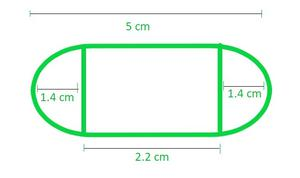Total Volume of one gulab jamun = Volume of two Hemisphere + Volume of cylinderπr3πr3 + πr2H

= πr2 (r + H)(taking π=)

= 25.05 cm3

Hence, volume of 45 gulab jamun = 45 × Volume of 1 gulab jamun

= 45 × 25.05

= 1127.28 cm3

As, gulab jamun contains sugar syrup up to about 30% of its volume

Sugar syrup in 45 gulab jamun = 30% of its total volume× 1127.28= 338.184 cm3

### Question 4. A pen stand made of wood is in the shape of a cuboid with four conical depressions to hold pens. The dimensions of the cuboid are 15 cm by 10 cm by 3.5 cm. The radius of each of the depressions is 0.5 cm and the depth is 1.4 cm. Find the volume of wood in the entire stand (see Fig.).

Solution:

Given:

Length of cuboid (l) = 15 cm

Breadth of cuboid (b) = 10 cm

Height of cuboid (h) = 3.5 cm

Radius of conical part (r) = 0.5 cm

Height of conical part (H) = 1.4 cm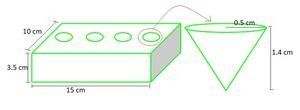Total Volume wood in the entire stand = Volume of Cuboid – Volume of four conical part

= (l × b × h) – 4 × (πr2H)

= (15 × 10 × 3.5) – (4 ×× 0.5 × 0.5 × 1.4)                           (taking π=)

= 525 – (1.466)

= 523.5333 cm3

### Question 5. A vessel is in the form of an inverted cone. Its height is 8 cm and the radius of its top, which is open, is 5 cm. It is filled with water up to the brim. When lead shots, each of which is a sphere of radius 0.5 cm are dropped into the vessel, one-fourth of the water flows out. Find the number of lead shots dropped in the vessel.

Solution:

Given:

Height of cone (h) = 8 cm

Radius of cone (r) = 5 cm

Radius of sphere (R)= 0.5 cm

Let, No. of sphere in cone = n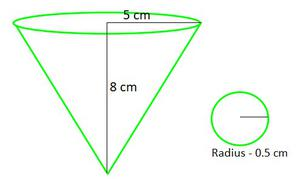Volume of water = Volume of coneπr2h× π × 5 × 5 × 8cm3

Volume of water flows out =(total volume of water)cm3

Hence, the volume of n spheres =cm3

Volume of each sphere =πr3× π × 0.5 × 0.5 ×0.5cm3

Hence, n =n =n = 100 spheres

### Question 6. A solid iron pole consists of a cylinder of height 220 cm and base diameter 24 cm, which is surmounted by another cylinder of height 60 cm and radius 8 cm. Find the mass of the pole, given that 1 cm3 of iron has approximately 8g mass. (Use π = 3.14)

Solution:

Given:

Height of large cylinder (H)= 220 cm

Radius of large cylinder (R)== 12 cm

Height of small cylinder (h)= 60 cm

Radius of small cylinder (r)= 8 cm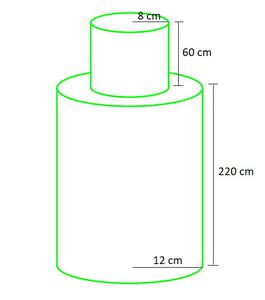Total Volume = Volume of large cylinder + Volume of small cylinder

= πR2H + πr2h

= π (R2H + r2h)

= 3.14 × ((12 × 12 × 220) + (8 × 8 × 60))                             (taking π=3.14)

= 3.14 (31680 + 3840)

= 111532.8 cm3

As given, Mass of 1cm3 = 8 g

Mass for 111532.8 cm3 = 8 × 111532.8 g

= 892,262.4 grams

= 892.2624 kg

### Question 7. A solid consisting of a right circular cone of height 120 cm and radius 60 cm standing on a hemisphere of radius 60 cm is placed upright in a right circular cylinder full of water such that it touches the bottom. Find the volume of water left in the cylinder, if the radius of the cylinder is 60 cm and its height is 180 cm.

Solution:

Given:

Height of cylinder (H)= 180 cm

Height of cone (h)= 180 – 60 = 120 cm

Radius of cone, cylinder and hemisphere (r) = 60 cm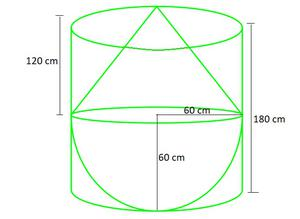Volume of water left in the cylinder = Volume of cylinder – (Volume of cone + Volume of hemisphere)

= πr2H – (πr2h +πr3)

= πr2 (H –h +r)(taking π=)× 60 × 60 × (100)

= 1131428.571 cm3

= 1.131 m3

Hence, the volume of water left in the cylinder = 1.131 m3

### Question 8. A spherical glass vessel has a cylindrical neck 8 cm long, 2 cm in diameter; the diameter of the spherical part is 8.5 cm. By measuring the amount of water it holds, a child finds its volume to be 345 cm3. Check whether she is correct, taking the above as the inside measurements, and π = 3.14.

Solution:

Given:

Height of cylinder (h)= 8 cm

Radius of cylinder (r) = 2/2 = 1 cm

Radius of sphere (R) =cm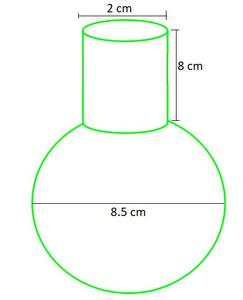Amount of water it can hold = Total Volume of this vessel

= Volume of cylinder + Volume of sphere

= πr2h +πR3= 8π + 102.35π

= 110.35π                       (taking π=3.14)

= 346.499 cm3

Volume measured by child = 345 cm3, which is INCORRECT

Correct volume = 346.5 cm3

My Personal Notes arrow_drop_up